# Simple Interest

#### Video Lesson on Simple Interest

• 1) Simple Interest: If the interest on a sum borrowed is reckoned uniformly, for a certain period, then it is called as simple interest. It is a quick method of calculating interest charged on a loan. Simple interest can be easily determined by multiplying the interest rate by principal by the number of periods.
• 2) Principal: It is the money borrowed or lent out for a certain period of time.
• 3) Interest: Rate of money paid regularly for using money on lent.
• 4) Total amount: Sum of principal and simple interest.

### Important Formulae

Simple interest on principal amount P for T years at the rate of R % is given as: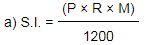### Quick tips and tricks

• 1) The rate of interest is always calculated per year unless specifically noted.
• 2) If in any numerical, the time given is specified in months, then convert it into years by simply dividing number of months by 12. If time is given in days, then convert days into year by dividing it with 365.
( Here rate of interest is given in percent and time in months (M))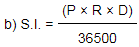( Here rate of interest is given in percent and time in days (D))
• 3) Principal is the amount deposited or borrowed, so do not get confused between such terms.
• 4) Simple Interest = Principle x Rate of Interest X Time
a) S.I. = PRT ------------------- (If rate of interest is in decimal form)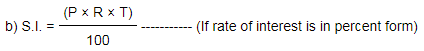• 5) If sum of money becomes (z times) in (T) years at simple interest, then rate of interest (R) can be calculated using the formula: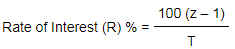• 6) If sum of money becomes (z times) at rate of interest (R) % per annum at simple interest, then time (T) can be calculated using the formula: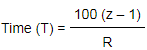### About us

EntryTest.com is a free service for students seeking successful career.CAT - College of Admission Tests. All rights reserved. College of Admission Tests Online Test Preparation The CAT Online